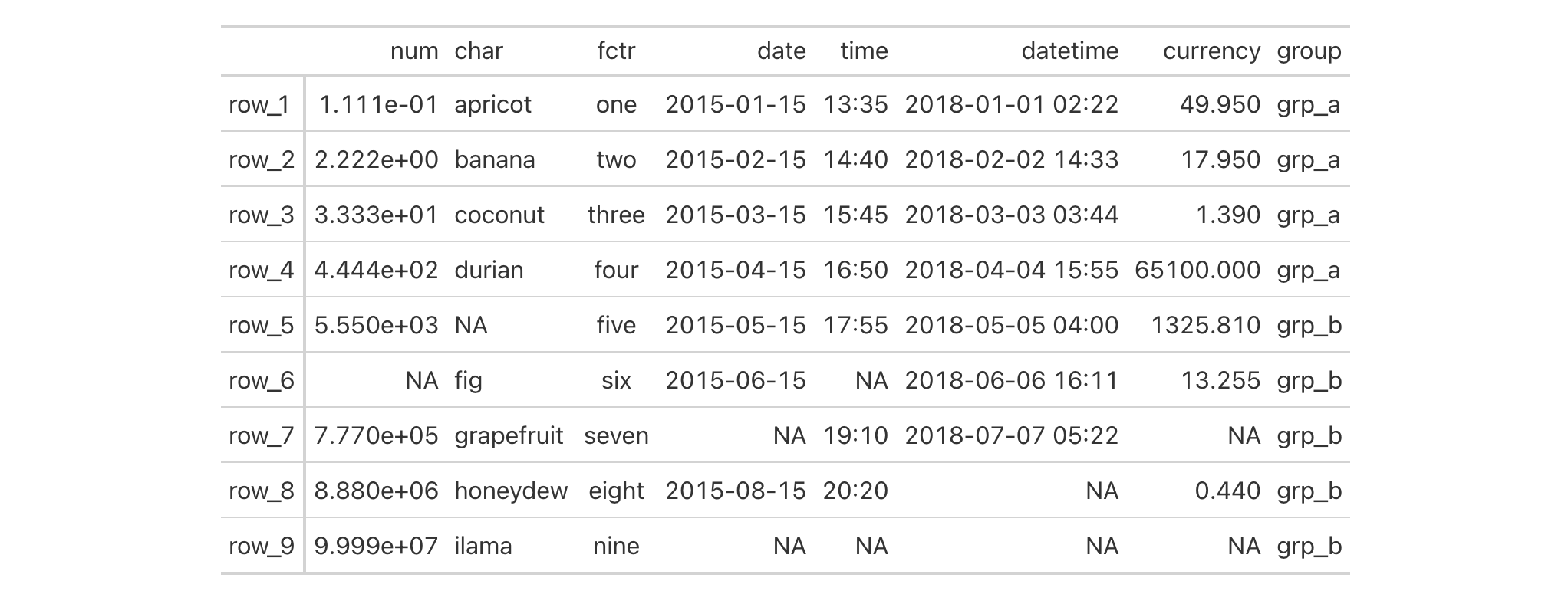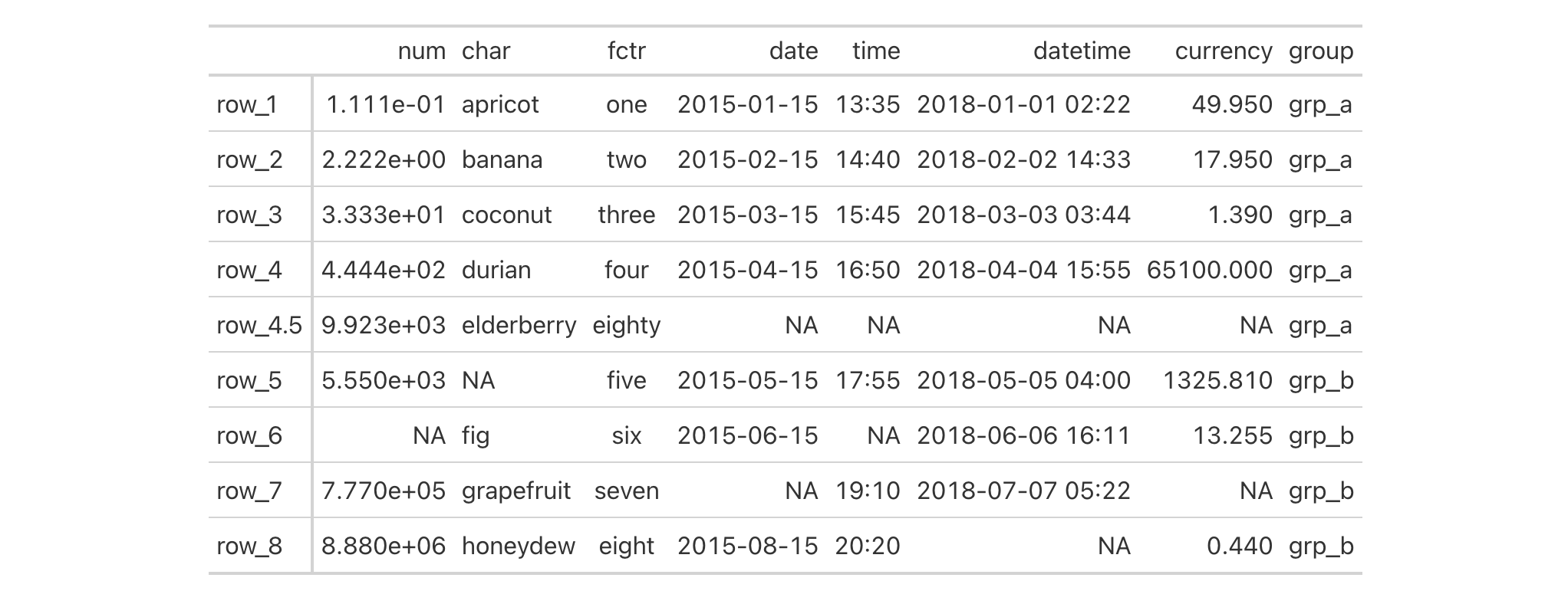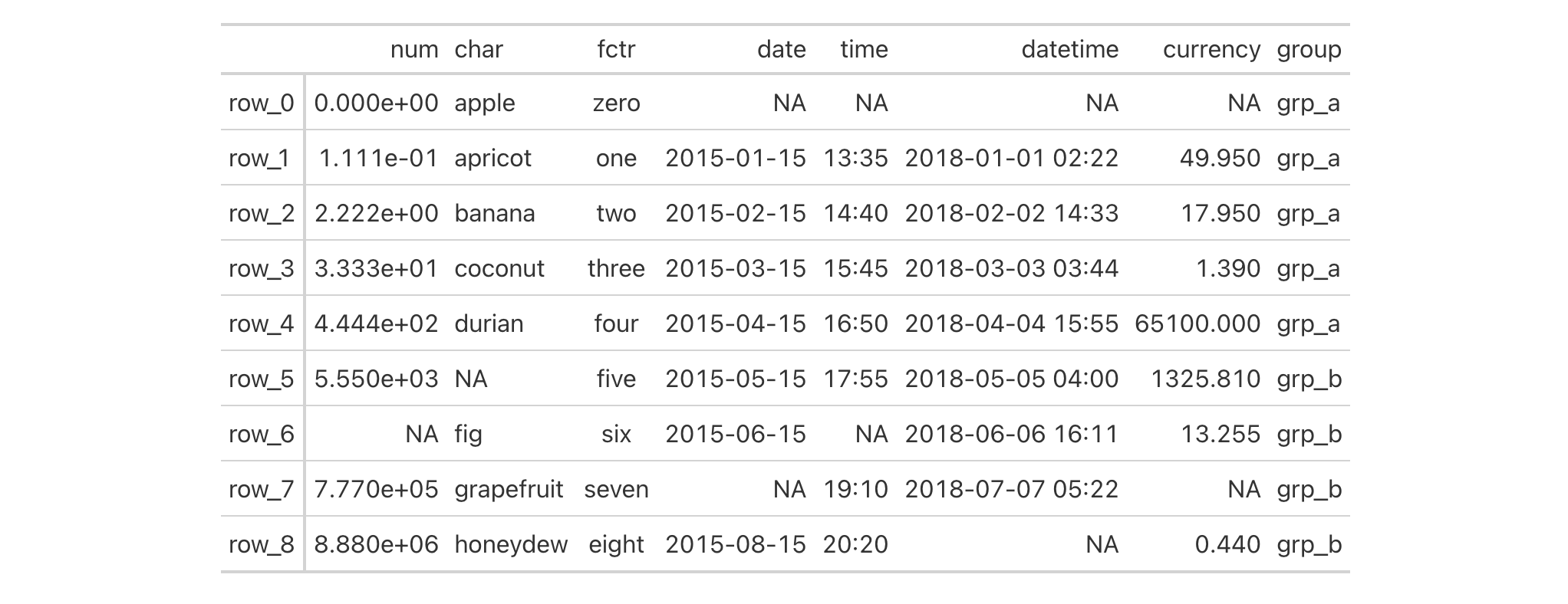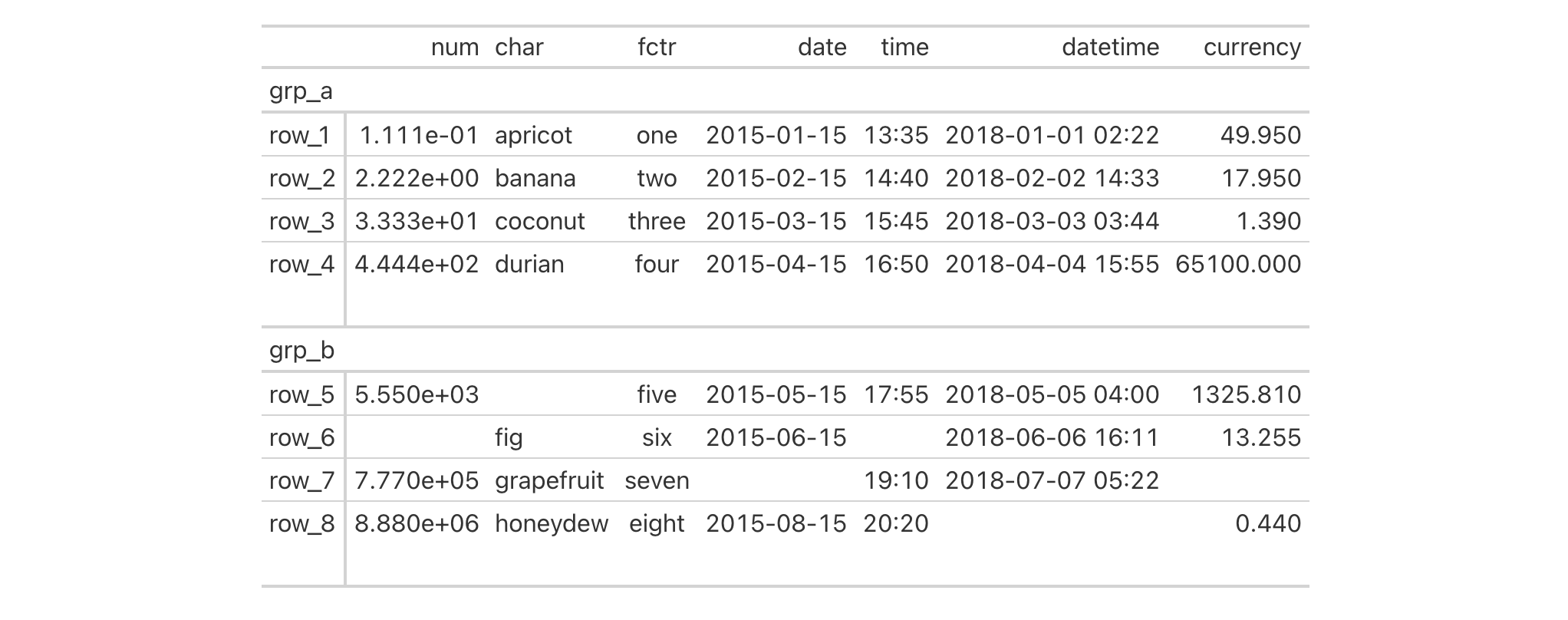It's possible to add new rows to your table with rows_add() by supplying the new row data through name-value pairs or two-sided formula expressions. The new rows are added to the bottom of the table by default but can be added internally though by using either the .before or .after arguments. If entirely empty rows need to be added, the .n_empty option provides a means to specify the number of blank (i.e., all NA) rows to be inserted into the table.

## Usage

rows_add(
.data,
...,
.list = list2(...),
.before = NULL,
.after = NULL,
.n_empty = NULL
)

## Arguments

.data

The gt table data object

obj:<gt_tbl> // required

This is the gt table object that is commonly created through use of the gt() function.

...

Cell data assignments

<multiple expressions> // (or, use .list)

Expressions for the assignment of cell values to the new rows by column name in .data. Name-value pairs, in the form of <column> = <value vector> will work, so long as the <column> value exists in the table. Two-sided formulas with column-resolving expressions (e.g, <expr> ~ <value vector>) can also be used, where the left-hand side corresponds to selections of columns. Column names should be enclosed in c() and select helpers like starts_with(), ends_with(), contains(), matches(), one_of(), and everything() can be used in the LHS. The length of the longest vector in <value vector> determines how many new rows will be added. Single values in <value vector> will be repeated down in cases where there are multiple rows to be added.

.list

Alternative to ...

<list of multiple expressions> // (or, use ...)

Allows for the use of a list as an input alternative to ....

.before, .after

Row used as anchor

<row-targeting expression> // default: NULL (optional)

A single row-resolving expression or row index an be given to either .before or .after. The row specifies where the new rows should be positioned among the existing rows in the input data table. While select helper functions such as starts_with() and ends_with() can be used for row targeting, it's recommended that a single row name or index be used. This is to ensure that exactly one row is provided to either of these arguments (otherwise, the function will be stopped). If nothing is provided for either argument then any new rows will be placed at the bottom of the table.

.n_empty

Number of empty rows to add

scalar<numeric|integer>(val>=0) // default: NULL (optional)

An option to add empty rows in lieu of rows containing data that would otherwise be supplied to ... or .list. If the option is taken, provide an integer value here.

## Value

An object of class gt_tbl.

## Targeting the row for insertion with .before or .after

The targeting of a row for insertion is done through the .before or .after arguments (only one of these options should be be used). This can be done in a variety of ways. If a stub is present, then we potentially have row identifiers. This is the ideal method to use for establishing a row target. We can use tidyselect-style expressions to target a row. It's also possible to use row indices (e.g., c(3, 5, 6)) though these index values must correspond to the row numbers of the input data (the indices won't necessarily match those of rearranged rows if row groups are present). One more type of expression is possible, an expression that takes column values (can involve any of the available columns in the table) and returns a logical vector.

## Examples

Let's make a simple gt table with the exibble dataset, using the row column for labels in the stub. We'll add a single row to the bottom of the table with rows_add(). With name-value pairs, it's possible to add values for the new body cells that correspond to columns available in the table. For any columns that are missed, the related body cells will receive NA values.

exibble |>
gt(rowname_col = "row") |>
row = "row_9",
num = 9.999E7,
char = "ilama",
fctr = "nine",
group = "grp_b"
)If you wanted to place a row somewhere in the middle of the table, we can use either of the .before or .after arguments in rows_add():

exibble |>
gt(rowname_col = "row") |>
row = "row_4.5",
num = 9.923E3,
char = "elderberry",
fctr = "eighty",
group = "grp_a",
.after = "row_4"
)Putting a row at the beginning requires the use of the .before argument. We can use an index value for the row as in .before = 1 for maximum easiness:

exibble |>
gt(rowname_col = "row") |>
row = "row_0",
num = 0,
char = "apple",
fctr = "zero",
group = "grp_a",
.before = 1
)Again with exibble, we can create an example where we insert 'spacer' rows. These are rows without any content and merely serve to add extra vertical space to the table in specific locations. In this case, we'll have a stub with row names and row groups (set up in the gt() call). The two rows being added will occupy the bottom row of each group. The only data defined for the two rows involves values for the row and group columns. It's important that the data for group uses the group names already present in the data ("grp_a" and "grp_b"). The corresponding values for row will be "row_a_end" and "row_b_end", these will be used later expressions for targeting the rows. Here's the code needed to generate spacer rows at the end of each row group:

exibble |>
gt(rowname_col = "row", groupname_col = "group") |>
row = c("row_a_end", "row_b_end"),
group = c("grp_a", "grp_b")
) |>
tab_style(
style = cell_borders(sides = "top", style = "hidden"),
locations = list(
cells_body(rows = ends_with("end")),
cells_stub(rows = ends_with("end"))
)
) |>
sub_missing(missing_text = "") |>
text_case_when(
grepl("end", x) ~ "",
.locations = cells_stub()
) |>
opt_vertical_padding(scale = 0.5)All missing values were substituted with an empty string (""), and that was done by using the sub_missing() function. We removed the top border of the new rows with a call to tab_style(), targeting those rows where the row labels end with "end". Finally, we get rid of the row labels with the use of the text_case_when() function, using a similar strategy of targeting the name of the row label.

Another application is starting from nothing (really just the definition of columns) and building up a table using several invocations of rows_add(). This might be useful in interactive or programmatic applications. Here's an example where two columns are defined with dplyr's tibble() function (and no rows are present initially); with two calls of rows_add(), two separate rows are added.

dplyr::tibble(
time = lubridate::POSIXct(),
event = character(0)
) |>
gt() |>
time = lubridate::ymd_hms("2022-01-23 12:36:10"),
event = "start"
) |>
time = lubridate::ymd_hms("2022-01-23 13:41:26"),
event = "completed"
)It's possible to use formula syntax in rows_add() to perform column resolution along with attaching values for new rows. If we wanted to use an equivalent value for multiple cells in a new row, a valid input would be in the form of <expr> ~ <value vector>. In the following example, we create a simple table with six columns (the rendered gt table displays four columns and a stub column since the group column is used for row group labels). Let's add a single row where some of the cell values added correspond to columns are resolved on the LHS of the formula expressions:

dplyr::tibble(
group = c("Group A", "Group B", "Group B"),
id = c("WG-025360", "WG-025361", "WG-025362"),
a = c(1, 6, 2),
b = c(2, 6, 2),
quantity_x = c(83.58, 282.71, 92.20),
quantity_y = c(36.82, 282.71, 87.34)
) |>
gt(rowname_col = "id", groupname_col = "group") |>
starts_with("gr") ~ "Group A",
id = "WG-025363",
c(a, b) ~ 5,
starts_with("quantity") ~ 72.63
)We can see that using starts_with("gr") yields a successful match to the group column with the tangible result being an addition of a row to the "Group A" group (the added row is the second one in the rendered gt table). Through the use of c(a, b), it was possible to add the value 5 to both the a and b columns. A similar approach was taken with adding the 72.63 value to the quantity_x and quantity_y columns though we used the starts_with("quantity") expression to get gt to resolve those two columns.

You can start with an empty table (i.e., no columns and no rows) and add one or more rows to it. In the completely empty table scenario, where you would use something like dplyr::tibble() or data.frame() with gt(), the first rows_add() could have rows of arbitrary width. In other words, you get to generate table columns (and rows) with a completely empty table via rows_add(). Here's an example of that:

gt(dplyr::tibble()) |>
msrp = c(29.95, 49.95, 79.95),
item = c("Klax", "Rez", "Ys"),
type = c("A", "B", "X")
) |>
msrp = 14.95,
item = "D",
type = "Z"
)In the above, three columns and three rows were generated. The second usage of rows_add() had to use of a subset of those columns (all three were used to create a complete, new row).

We can also start with a virtually empty table: one that has columns but no actual rows. With this type of multi-column, zero-row table, one needs to use a subset of the columns when generating new rows through rows_add().

dplyr::tibble(
msrp = numeric(0),
item = character(0),
type = character(0)
) |>
gt() |>
msrp = c(29.95, 49.95, 79.95, 14.95),
item = c("Klax", "Rez", "Ys", "D"),
type = c("A", "B", "X", "Z")
) |>
genre = c("puzzle", "action", "RPG", "adventure")
) |>
fmt_currency() |>
cols_move_to_end(columns = msrp)6-4

## Function Introduced

v0.10.0 (October 7, 2023)

Other row addition/modification functions: grand_summary_rows(), row_group_order(), summary_rows()